﻿ Warming up for our 13th year

 Like   Tweet
 /* styles */ Dear Readers, Finally spring is with us and the Dayton Literary Peace Prize is warming up for its 13th year. We have exciting news and events for now and later to fill your calendar. Please join us on May 23rd to welcome Gilbert King and his new book, Beneath a Ruthless Sun. The 2013 finalists will not be announced for several months, but you have time to catch up with some of our former winners. Our website is a rich resource for finding those summer books you have been meaning to read—Colm Tóibín, Patricia Engel, and David Wood will be returning for this year’s gala, and Ben Rawlence will be coming to Dayton for the first time.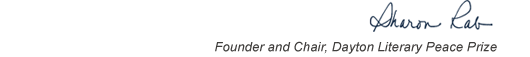table div table+table+table+table div table{width:100%;padding:0}table div table+table+table+table div table img{width:96.23%;padding:0;float:none}table div table+table+table+table div table td{width:100%;padding:0 1.88% 18px}/* styles */# Gilbert King at Books & Co.

 table div table+table+table+table+table+table div table{width:100%;padding:0}table div table+table+table+table+table+table div table img{width:96.23%;padding:0;float:none}table div table+table+table+table+table+table div table td{width:100%;padding:0 1.88% 18px}/* styles */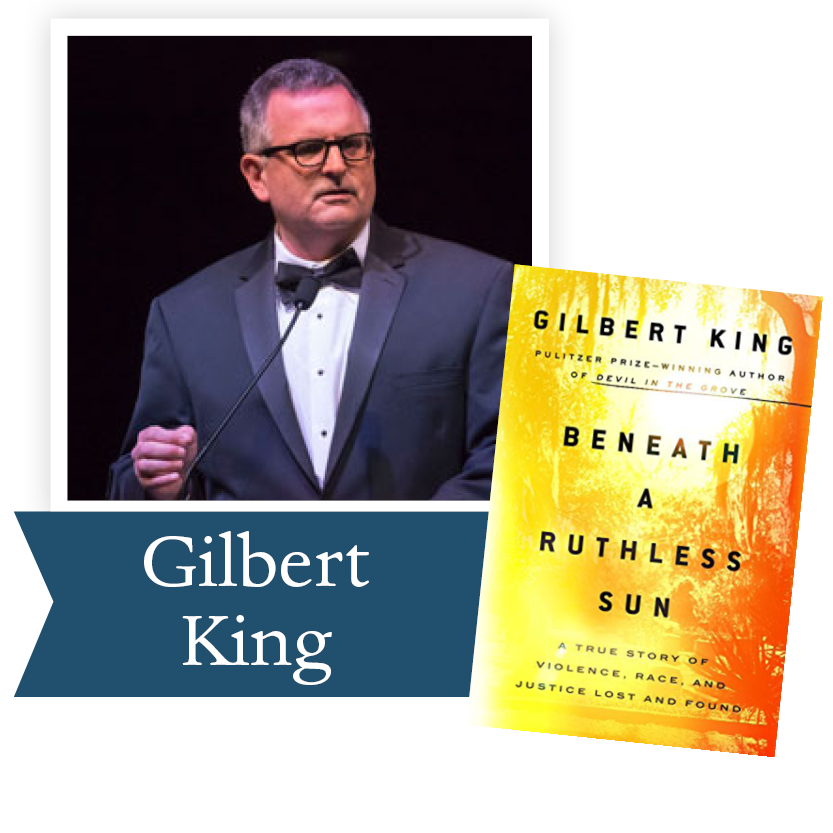Photo credit: Andy Snow

Gilbert King, the 2013 Dayton Literary Peace Prize Nonfiction Runner-up for Devil in the Grove, will introduce his newest nonfiction book on Wednesday, May 23rd at 7 pm at Books & Co. at The Greene, 4453 Walnut Street, Dayton. His new book, Beneath a Ruthless Sun: A True Story of Violence, Race, and Justice Lost and Found, is a gripping true-crime tale that raises themes that haunt us still today: justice perverted by bigotry and the force of white privilege; wrongful incarceration; and the power of the free press.

 table div table+table+table+table+table+table+table+table div table{width:100%;padding:0}table div table+table+table+table+table+table+table+table div table img{width:96.23%;padding:0;float:none}table div table+table+table+table+table+table+table+table div table td{width:100%;padding:0 1.88% 18px}/* styles */# The Holbrooke Society of the Dayton Literary Peace Prize Foundation

 table div table+table+table+table+table+table+table+table+table+table div table{width:100%;padding:0}table div table+table+table+table+table+table+table+table+table+table div table img{width:96.23%;padding:0;float:none}table div table+table+table+table+table+table+table+table+table+table div table td{width:100%;padding:0 1.88% 18px}/* styles */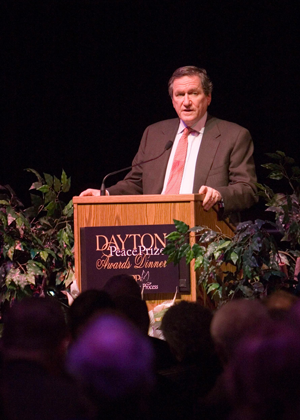In addition to continuing the current quality of events and ensuring the sustainability of the organization, the DLPP Foundation is dedicated to expanding educational programming and outreach, which will necessitate increased funding and staff. At the heart of the sustainability plan is the creation of The Holbrooke Society of the Dayton Literary Peace Prize Foundation, an annual giving society for individuals. Kati Marton, Ambassador Holbrooke’s widow, has given us her blessing in establishing this society.

The Holbrooke Society of the Dayton Literary Peace Prize Foundation
The Accords Circle of The Holbrooke Society
(\$5,000 - \$9,999)

• \$5,000 minimum annual contribution (not associated with a table purchase or a sponsorship)
• Invitation for two to the Saturday evening dinner
• Two tickets to the Sunday evening awards gala
• A set of the prize-winning books
• Invitation for two to a special reception/dinner associated with the Authors’ Series
• Recognition in the program
• Invitations for two to private receptions for additional DLPP events

The Laureate Circle of The Holbrooke Society
(\$10,000+)

All of the benefits of The Holbrooke Society plus additional exclusive events with prize-winning authors and significant writers associated with the DLPP.

 table div table+table+table+table+table+table+table+table+table+table+table+table div table{width:100%;padding:0}table div table+table+table+table+table+table+table+table+table+table+table+table div table img{width:96.23%;padding:0;float:none}table div table+table+table+table+table+table+table+table+table+table+table+table div table td{width:100%;padding:0 1.88% 18px}/* styles */# 2018 Dayton Literary Peace Prize Updates

 table div table+table+table+table+table+table+table+table+table+table+table+table+table+table div table{width:100%;padding:0}table div table+table+table+table+table+table+table+table+table+table+table+table+table+table div table img{width:96.23%;padding:0;float:none}table div table+table+table+table+table+table+table+table+table+table+table+table+table+table div table td{width:100%;padding:0 1.88% 18px}/* styles */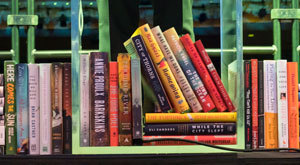Photo credit: Andy Snow

The Dayton Literary Peace Prize received a record number of 106 submissions this year! Judges are currently working on the first round of evaluations to determine the finalists. As of this year, 224 publishing houses have nominated books for the DLPP.

 table div table+table+table+table+table+table+table+table+table+table+table+table+table+table+table+table div table{width:100%;padding:0}table div table+table+table+table+table+table+table+table+table+table+table+table+table+table+table+table div table img{width:96.23%;padding:0;float:none}table div table+table+table+table+table+table+table+table+table+table+table+table+table+table+table+table div table td{width:100%;padding:0 1.88% 18px}/* styles */# Fly with the Doves

 table div table+table+table+table+table+table+table+table+table+table+table+table+table+table+table+table+table+table div table{width:100%;padding:0}table div table+table+table+table+table+table+table+table+table+table+table+table+table+table+table+table+table+table div table img{width:96.23%;padding:0;float:none}table div table+table+table+table+table+table+table+table+table+table+table+table+table+table+table+table+table+table div table td{width:100%;padding:0 1.88% 18px}/* styles */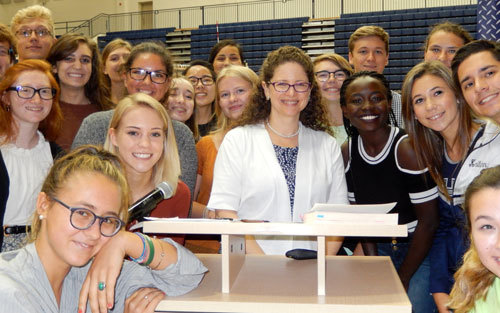Karima Bennoune at Author Series event with students

The Dayton Literary Peace Prize Fly with the Doves Book Circle was formed to allow the DLPP to donate winning and nominated books to schools, universities, and libraries. With memberships beginning at \$25.00, supporting members provide the funding to share these powerful works of literature both locally and around the world.

In 2018, the Dayton Literary Peace Prize Fly with the Doves Book Circle will complete the collection of DLPP books for the National Library at Sarajevo and will help local high schools secure books for students participating in the DLPP Author Series in October.

 table div table+table+table+table+table+table+table+table+table+table+table+table+table+table+table+table+table+table+table+table div table{width:100%;padding:0}table div table+table+table+table+table+table+table+table+table+table+table+table+table+table+table+table+table+table+table+table div table img{width:96.23%;padding:0;float:none}table div table+table+table+table+table+table+table+table+table+table+table+table+table+table+table+table+table+table+table+table div table td{width:100%;padding:0 1.88% 18px}/* styles */table div table+table+table+table+table+table+table+table+table+table+table+table+table+table+table+table+table+table+table+table+table+table div table{width:100%;padding:0}table div table+table+table+table+table+table+table+table+table+table+table+table+table+table+table+table+table+table+table+table+table+table div table img{width:96.23%;padding:0;float:none}table div table+table+table+table+table+table+table+table+table+table+table+table+table+table+table+table+table+table+table+table+table+table div table td{width:100%;padding:0 1.88% 18px}/* styles */table div table+table+table+table+table+table+table+table+table+table+table+table+table+table+table+table+table+table+table+table+table+table+table+table+table+table div table{width:100%;padding:0}table div table+table+table+table+table+table+table+table+table+table+table+table+table+table+table+table+table+table+table+table+table+table+table+table+table+table div table img{width:96.23%;padding:0;float:none}table div table+table+table+table+table+table+table+table+table+table+table+table+table+table+table+table+table+table+table+table+table+table+table+table+table+table div table td{width:100%;padding:0 1.88% 18px}/* styles */